Would you like to see this cheatsheet in your native language? You can help us translating it on GitHub!

# Reflex-based models with Machine Learning

By Afshine Amidi and Shervine Amidi

## Linear predictors

In this section, we will go through reflex-based models that can improve with experience, by going through samples that have input-output pairs.

Feature vector ― The feature vector of an input $x$ is noted $\phi(x)$ and is such that:

$\boxed{\phi(x)=\left[\begin{array}{c}\phi_1(x)\\\vdots\\\phi_d(x)\end{array}\right]\in\mathbb{R}^d}$

Score ― The score $s(x,w)$ of an example $(\phi(x),y) \in \mathbb{R}^d \times \mathbb{R}$ associated to a linear model of weights $w \in \mathbb{R}^d$ is given by the inner product:

$\boxed{s(x,w)=w\cdot\phi(x)}$

### Classification

Linear classifier ― Given a weight vector $w\in\mathbb{R}^d$ and a feature vector $\phi(x)\in\mathbb{R}^d$, the binary linear classifier $f_w$ is given by:

$\boxed{f_w(x)=\textrm{sign}(s(x,w))=\left\{ \begin{array}{cr} +1 & \textrm{if }w\cdot\phi(x)>0\\ -1 & \textrm{if }w\cdot\phi(x)< 0\\ ? & \textrm{if }w\cdot\phi(x)=0 \end{array}\right.}$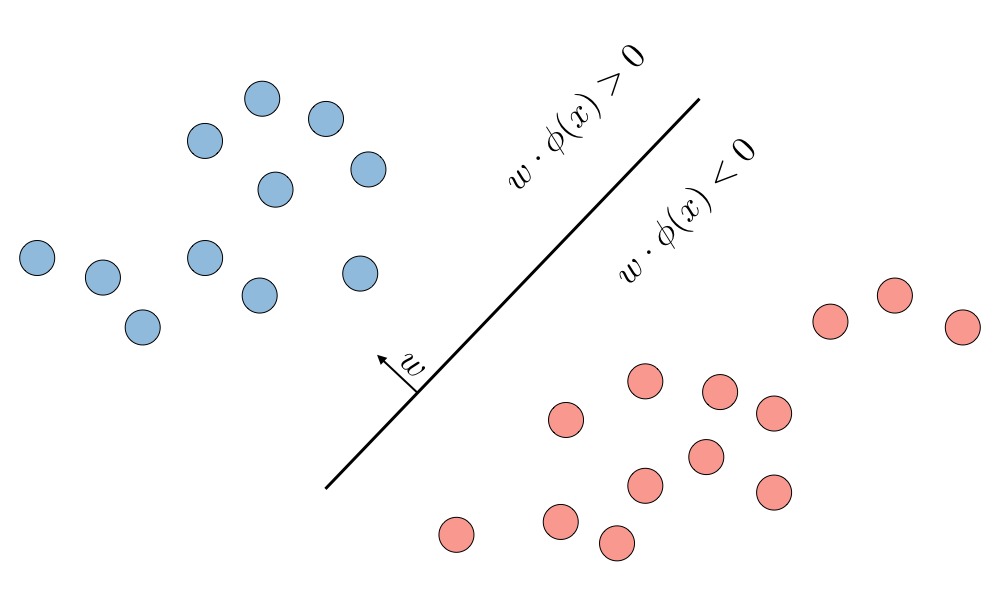Margin ― The margin $m(x,y,w) \in \mathbb{R}$ of an example $(\phi(x),y) \in \mathbb{R}^d \times \{-1,+1\}$ associated to a linear model of weights $w\in \mathbb{R}^d$ quantifies the confidence of the prediction: larger values are better. It is given by:

$\boxed{m(x,y,w)=s(x,w)\times y}$

### Regression

Linear regression ― Given a weight vector $w\in\mathbb{R}^d$ and a feature vector $\phi(x)\in\mathbb{R}^d$, the output of a linear regression of weights $w$ denoted as $f_w$ is given by:

$\boxed{f_w(x)=s(x,w)}$

Residual ― The residual $\textrm{res}(x,y,w) \in \mathbb{R}$ is defined as being the amount by which the prediction $f_w(x)$ overshoots the target $y$:

$\boxed{\textrm{res}(x,y,w)=f_w(x)-y}$

## Loss minimization

Loss function ― A loss function $\textrm{Loss}(x,y,w)$ quantifies how unhappy we are with the weights $w$ of the model in the prediction task of output $y$ from input $x$. It is a quantity we want to minimize during the training process.

Classification case - The classification of a sample $x$ of true label $y\in \{-1,+1\}$ with a linear model of weights $w$ can be done with the predictor $f_w(x) \triangleq \textrm{sign}(s(x,w))$. In this situation, a metric of interest quantifying the quality of the classification is given by the margin $m(x,y,w)$, and can be used with the following loss functions:

 Name Zero-one loss Hinge loss Logistic loss $\textrm{Loss}(x,y,w)$ $1_{\{m(x,y,w) \leqslant 0\}}$ $\max(1-m(x,y,w), 0)$ $\log(1+e^{-m(x,y,w)})$ Illustration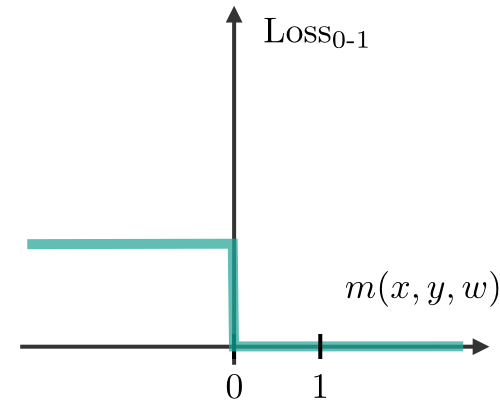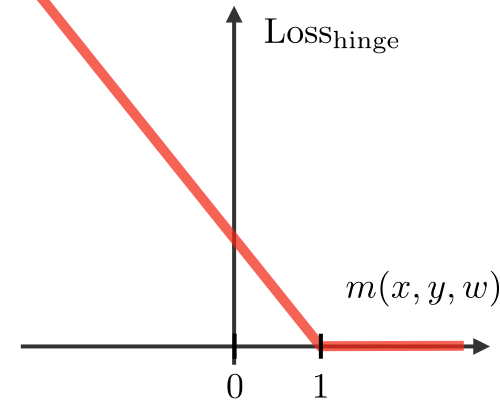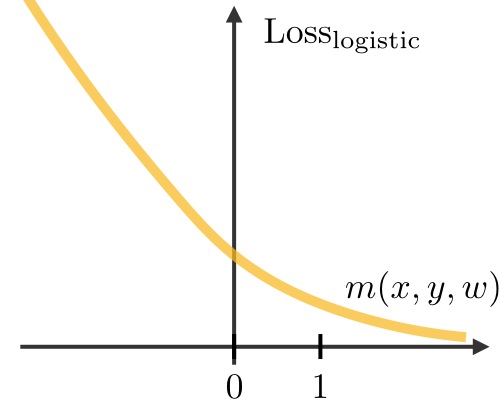Regression case - The prediction of a sample $x$ of true label $y \in \mathbb{R}$ with a linear model of weights $w$ can be done with the predictor $f_w(x) \triangleq s(x,w)$. In this situation, a metric of interest quantifying the quality of the regression is given by the margin $\textrm{res}(x,y,w)$ and can be used with the following loss functions:

 Name Squared loss Absolute deviation loss $\textrm{Loss}(x,y,w)$ $(\textrm{res}(x,y,w))^2$ $|\textrm{res}(x,y,w)|$ Illustration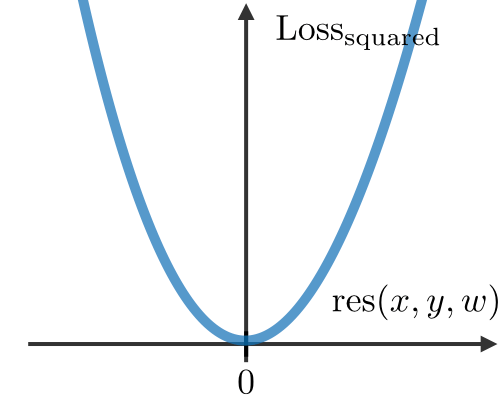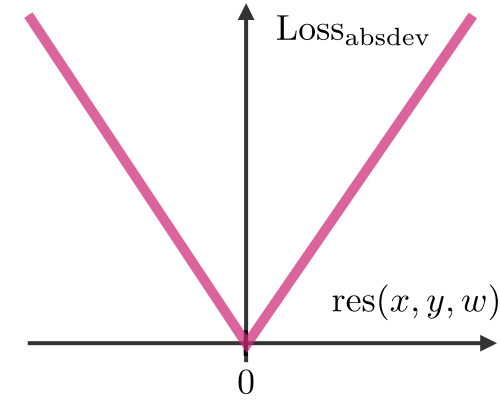Loss minimization framework ― In order to train a model, we want to minimize the training loss is defined as follows:

$\boxed{\textrm{TrainLoss}(w)=\frac{1}{|\mathcal{D}_{\textrm{train}}|}\sum_{(x,y)\in\mathcal{D}_{\textrm{train}}}\textrm{Loss}(x,y,w)}$

## Non-linear predictors

$k$-nearest neighbors ― The $k$-nearest neighbors algorithm, commonly known as $k$-NN, is a non-parametric approach where the response of a data point is determined by the nature of its $k$ neighbors from the training set. It can be used in both classification and regression settings.Remark: the higher the parameter $k$, the higher the bias, and the lower the parameter $k$, the higher the variance.

Neural networks ― Neural networks are a class of models that are built with layers. Commonly used types of neural networks include convolutional and recurrent neural networks. The vocabulary around neural networks architectures is described in the figure below:By noting $i$ the $i^{th}$ layer of the network and $j$ the $j^{th}$ hidden unit of the layer, we have:

$\boxed{z_j^{[i]}={w_j^{[i]}}^Tx+b_j^{[i]}}$

where we note $w$, $b$, $x$, $z$ the weight, bias, input and non-activated output of the neuron respectively.

For a more detailed overview of the concepts above, check out the Supervised Learning cheatsheets!

## Stochastic gradient descent

Gradient descent ― By noting $\eta\in\mathbb{R}$ the learning rate (also called step size), the update rule for gradient descent is expressed with the learning rate and the loss function $\textrm{Loss}(x,y,w)$ as follows:

$\boxed{w\longleftarrow w-\eta\nabla_w \textrm{Loss}(x,y,w)}$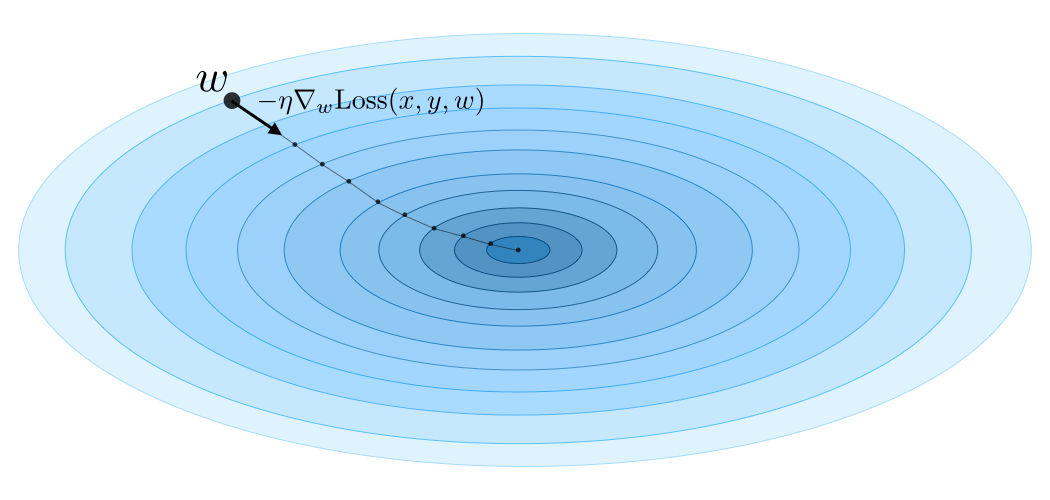Stochastic updates ― Stochastic gradient descent (SGD) updates the parameters of the model one training example $(\phi(x),y)\in\mathcal{D}_{\textrm{train}}$ at a time. This method leads to sometimes noisy, but fast updates.

Batch updates ― Batch gradient descent (BGD) updates the parameters of the model one batch of examples (e.g. the entire training set) at a time. This method computes stable update directions, at a greater computational cost.

## Fine-tuning models

Hypothesis class ― A hypothesis class $\mathcal{F}$ is the set of possible predictors with a fixed $\phi(x)$ and varying $w$:

$\boxed{\mathcal{F}=\left\{f_w:w\in\mathbb{R}^d\right\}}$

Logistic function ― The logistic function $\sigma$, also called the sigmoid function, is defined as:

$\boxed{\forall z\in]-\infty,+\infty[,\quad\sigma(z)=\frac{1}{1+e^{-z}}}$

Remark: we have $\sigma'(z)=\sigma(z)(1-\sigma(z))$.

Backpropagation ― The forward pass is done through $f_i$, which is the value for the subexpression rooted at $i$, while the backward pass is done through $g_i=\frac{\partial\textrm{out}}{\partial f_i}$ and represents how $f_i$ influences the output.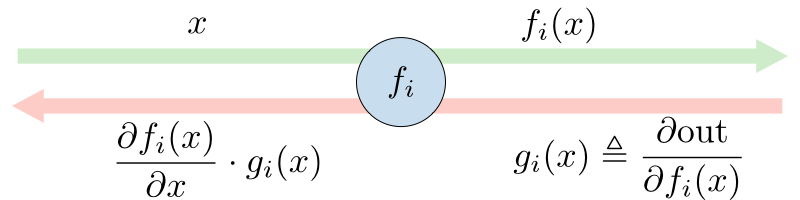Approximation and estimation error ― The approximation error $\epsilon_\text{approx}$ represents how far the entire hypothesis class $\mathcal{F}$ is from the target predictor $g^*$, while the estimation error $\epsilon_{\text{est}}$ quantifies how good the predictor $\hat{f}$ is with respect to the best predictor $f^{*}$ of the hypothesis class $\mathcal{F}$.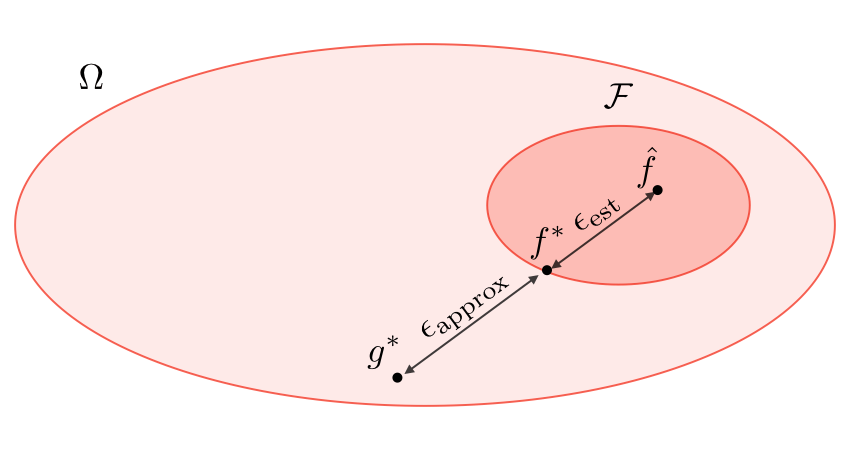Regularization ― The regularization procedure aims at avoiding the model to overfit the data and thus deals with high variance issues. The following table sums up the different types of commonly used regularization techniques:

 LASSO Ridge Elastic Net • Shrinks coefficients to 0• Good for variable selection Makes coefficients smaller Tradeoff between variable selection and small coefficients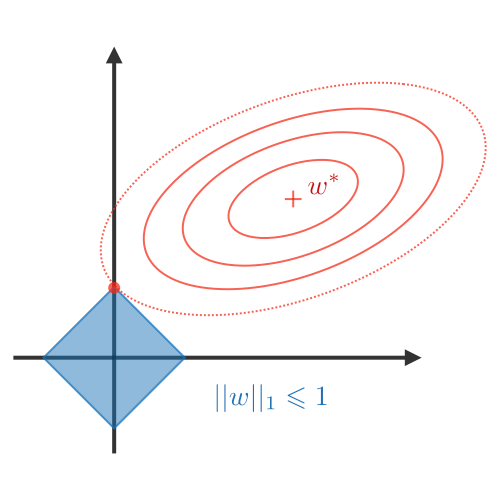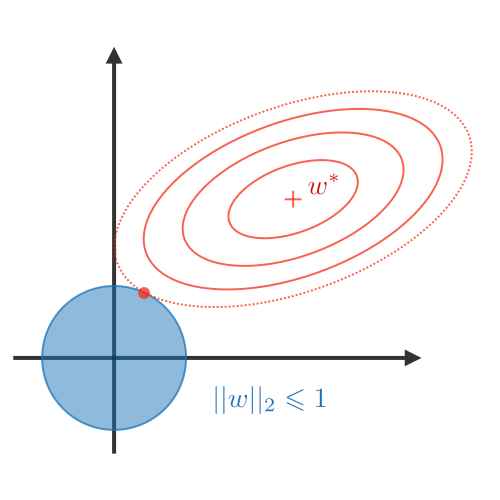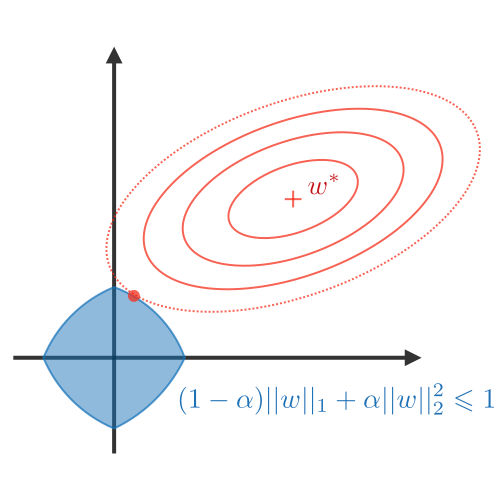$...+\lambda||w||_1$$\lambda\in\mathbb{R} ...+\lambda||w||_2^2$$\lambda\in\mathbb{R}$ $...+\lambda\Big[(1-\alpha)||w||_1+\alpha||w||_2^2\Big]$$\lambda\in\mathbb{R},\alpha\in[0,1]$

Hyperparameters ― Hyperparameters are the properties of the learning algorithm, and include features, regularization parameter $\lambda$, number of iterations $T$, step size $\eta$, etc.

Sets vocabulary ― When selecting a model, we distinguish 3 different parts of the data that we have as follows:

 Training set Validation set Testing set • Model is trained • Usually 80% of the dataset • Model is assessed • Usually 20% of the dataset • Also called hold-out or development set • Model gives predictions • Unseen data

Once the model has been chosen, it is trained on the entire dataset and tested on the unseen test set. These are represented in the figure below:For a more detailed overview of the concepts above, check out the Machine Learning tips and tricks cheatsheets!

## Unsupervised Learning

The class of unsupervised learning methods aims at discovering the structure of the data, which may have of rich latent structures.

### $k$-means

Clustering ― Given a training set of input points $\mathcal{D}_{\textrm{train}}$, the goal of a clustering algorithm is to assign each point $\phi(x_i)$ to a cluster $z_i\in\{1,...,k\}$.

Objective function ― The loss function for one of the main clustering algorithms, $k$-means, is given by:

$\boxed{\textrm{Loss}_{\textrm{k-means}}(x,\mu)=\sum_{i=1}^n||\phi(x_i)-\mu_{z_i}||^2}$

Algorithm ― After randomly initializing the cluster centroids $\mu_1,\mu_2,...,\mu_k\in\mathbb{R}^n$, the $k$-means algorithm repeats the following step until convergence:

$\boxed{z_i=\underset{j}{\textrm{arg min}}||\phi(x_i)-\mu_j||^2}\quad\textrm{and}\quad\boxed{\mu_j=\frac{\displaystyle\sum_{i=1}^m1_{\{z_i=j\}}\phi(x_i)}{\displaystyle\sum_{i=1}^m1_{\{z_i=j\}}}}$### Principal Component Analysis

Eigenvalue, eigenvector ― Given a matrix $A\in\mathbb{R}^{n\times n}$, $\lambda$ is said to be an eigenvalue of $A$ if there exists a vector $z\in\mathbb{R}^n\backslash\{0\}$, called eigenvector, such that we have:

$\boxed{Az=\lambda z}$

Spectral theorem ― Let $A\in\mathbb{R}^{n\times n}$. If $A$ is symmetric, then $A$ is diagonalizable by a real orthogonal matrix $U\in\mathbb{R}^{n\times n}$. By noting $\Lambda=\textrm{diag}(\lambda_1,...,\lambda_n)$, we have:

$\boxed{\exists\Lambda\textrm{ diagonal},\quad A=U\Lambda U^T}$

Remark: the eigenvector associated with the largest eigenvalue is called principal eigenvector of matrix $A$.

Algorithm ― The Principal Component Analysis (PCA) procedure is a dimension reduction technique that projects the data on $k$ dimensions by maximizing the variance of the data as follows:
- Step 1: Normalize the data to have a mean of 0 and standard deviation of 1.

$\boxed{\phi_j(x_i)\leftarrow\frac{\phi_j(x_i)-\mu_j}{\sigma_j}}\quad\mbox{where}\quad\boxed{\mu_j = \frac{1}{m}\sum_{i=1}^m\phi_j(x_i)}\quad\mbox{and}\quad\boxed{\sigma_j^2=\frac{1}{m}\sum_{i=1}^m(\phi_j(x_i)-\mu_j)^2}$

- Step 2: Compute $\displaystyle\Sigma=\frac{1}{m}\sum_{i=1}^m\phi(x_i){\phi(x_i)}^T\in\mathbb{R}^{n\times n}$, which is symmetric with real eigenvalues.
- Step 3: Compute $u_1, ..., u_k\in\mathbb{R}^n$ the $k$ orthogonal principal eigenvectors of $\Sigma$, i.e. the orthogonal eigenvectors of the $k$ largest eigenvalues.
- Step 4: Project the data on $\textrm{span}_\mathbb{R}(u_1,...,u_k)$.

This procedure maximizes the variance among all $k$-dimensional spaces.For a more detailed overview of the concepts above, check out the Unsupervised Learning cheatsheets!